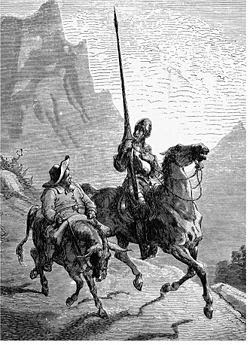## Thursday, February 5, 2015

### The monkey pounding on a typewriter

In connection with the fine tuning problem, the argument of the typist monkey is often used as an illustration that even very unlikely events can occur spontaneously. Depending on the author of the quote, the text supposedly written by the monkey can be the complete works of Shakespeare, Don Quixote, or even a shorter and less specific work. For instance, John Leslie, in his book Universes (Chapter One), writes:
Our universe can indeed look as if designed. In reality, though,  it may be merely the sort of thing to be expected sooner or later. Given sufficient many years with a typewriter, even a monkey would produce a sonnet.
Really? ¿A sufficient number of years? How many? Have you tried to calculate it?
Let us simplify the problem. We are going to ask the monkey to type, by pounding randomly the keys of the typewriter, something much shorter than a work by Shakespeare, or even a sonnet. We will just ask for the first sentence in Don Quixote:
En un lugar de la Mancha, de cuyo nombre no quiero acordarme, no ha mucho tiempo que vivía un hidalgo de los de lanza en astillero, adarga antigua, rocín flaco y galgo corredor.
We assume further that the typewriter has only the minimum number of keys: 27 letters, comma, period and blank space. To simplify even more, we accept a text written in lower case. Can we estimate how long it would take the monkey to write this text, by pounding the keys at random?Drawing by Gustavo Doré
Let's see. The probability that a certain text of 177 characters is generated by banging randomly on 30 keys (we assume that the probability of the monkey pressing any key is the same) is 30-177»3.5´10-262.
Assume that the monkey is able to type 177 characters per minute. In that case, what he produces every minute can be considered an attempt to reproduce the first sentence of Don Quixote. Assuming (a big assumption) that no text is repeated, that all the attempts are different, how long would be needed to produce all the possible variations of the characters in the indicated text, one of which is this piece of Don Quixote? It is easy to see that it would take 2.8´10261 minutes (the inverse of the number of variations), equivalent to about 5.3´10255 years, i.e., 3.8´10245 times the age of the universe (a 3 and an 8 followed by 244 zeros).
The conclusion is obvious: however many years we left the monkey with the typewriter, it is not reasonable to think that it would be able to type that small piece of Don Quixote, as it would die much earlier. In fact, if we force the poor monkey to pound all the time on the typewriter, with no time to eat or sleep, its life expectancy will be dramatically reduced.
Yes, I know, the monkey is metaphorical, and the objective of the problem is to show that, given enough time, however long, even extremely improbable events may happen (those with a very small probability, greater than zero). But look at the question from the opposite side. If we find the text quoted above, which of the following two hypotheses should be considered more rational?
That the text was written by a person with a certain intention.

Or that the text arose by chance, as though it had been produced by a monkey pounding on a typewriter.

The same post in Spanish
Thematic thread on Multiverse and Fine Tuning: Preceding Next
Thematic Thread on Statistics: Previous Next
Manuel Alfonseca
(For Fonch)

1.Excellent post!! Elena from the USA

2.Thank you so much for the interesting article on a suggestion I've always found unsound.

3.Thanks. I have a couple of things to add to the post:

1. An event may have a very low probability, but its occurrence may be certain. So, if you buy a single ticket for a lottery with 100.000 different numbers, your probability of winning is 10^-5, but if all the numbers are sold, someone will win the first prize anyway, even with such a low probability. This happens because there are many people playing, and if a number is sold, nobody else can buy it.

Does this happen in the monkey case? Not at all. Let us assume that the probability of a text being written were 10^-500, and that there are 10^500 monkeys pounding on typewriters in parallel. Would the text in question be sure to written in this situation? No, because we couldn't assume (as I did in the post) that two monkeys will always write different texts. In fact, the number of repetitions could be very large, without any limitation. Therefore it would not be certain that the text in don Quixote would ever be written.

2. The multiverse hypothesis has been introduced to solve the fine tuning problem, but it doesn't. String theory, for instance, postulates the existence of 10^500 possible different universes. But even if there were 10^500 universes in existence, we wouldn't be able to assure that there were no repetitions, therefore we would be in the same situation as the 10^500 monkeys pounding on typewriters. Therefore those multiverses do not solve the fine tuning problem. To do that, the only way is postulating the existence of an infinite number of universes (as Tegmark does). But this introduces other problems, as I have pointed in previous posts in this blog.